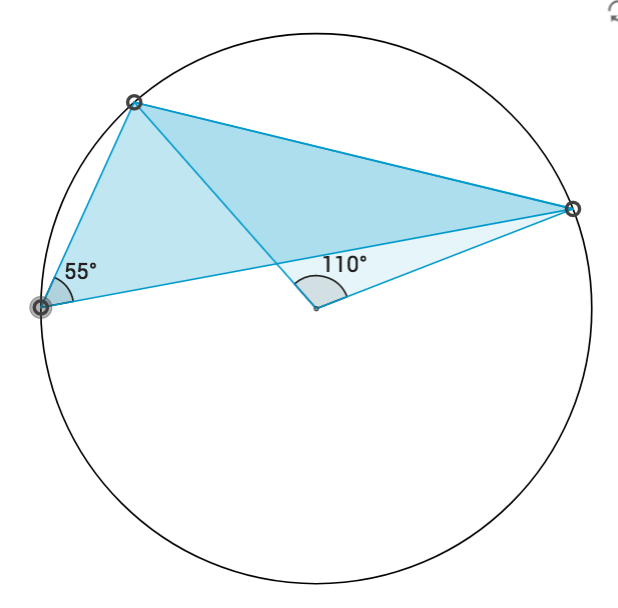# Angle at the Center of a Circle

This theorem, written down by Euclid about 2300 years ago, says something along the lines of:

“consider a chord drawn in a circle. If the chord subtends an angleat the center, then it subtends an angleat any point on the circumference on the major segment”.

Move the points on the circumference around to build an appreciation of this theorem.

Now let’s prove the theorem. Here is a diagram to get started with.Using the diagram, prove thatFirst Hint

Second hint

Third hint

Here is this proof written out.

Euclid’s proof is slightly different. Euclid uses the theorem about the exterior angle of a triangle – the exterior angle of a triangle is equal to the sum of the opposite sides.

Notice that either of these proofs work well when the center of the circle is within the triangle. Both need to be tweeked when the center is not in the triangle, for example:That’s either yours or tomorrow’s project to produce those other proofs…

Done here! Let’s go back to the triangles menu.